# NCERT Solutions for Chapter 1 Knowing our Numbers Class 6 Maths

###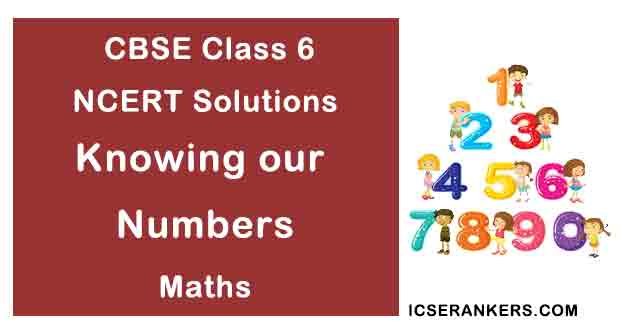Knowing our Numbers Questions and Answers

 Chapter Name Knowing our Numbers NCERT Solutions Class CBSE Class 6 Textbook Name Maths Related Readings NCERT Solutions for Class 6NCERT Solutions for Class 6 Maths Summary of Knowing our Numbers

Exercise 1.1

Question 1: Fill in the blanks:

(a) 1 lakh = ______ ten thousand

(b) 1 million = ______ hundred thousand

(c) 1 crore = ______ ten lakh

(d) 1 crore = ______ million

(e) 1 million = ______ lakh

Solution

(a) 10

(b) 10

(c) 10

(d) 10

(e) 10

Question 2: Place commas correctly and write the numerals:

(a) Seventy-three lakh seventy-five thousand three hundred seven.

(b) Nine crore five lakh forty-one.

(c) Seven crore fifty-two lakh twenty-one thousand three hundred two.

(d) Fifty-eight million four hundred twenty-three thousand two hundred two.

(e) Twenty-three lakh thirty thousand ten.

Solution

(a) 73,75,307

(b) 9,05,00,041

(c) 7,52,21,302

(d) 58,423,202

(e) 23,30,010

Question 3: Insert commas suitable and write the names according to Indian system of numeration:

(a) 87595762

(b) 8546283

(c) 99900046

(d) 98432701

Solution

(a) 8,75,95,762

Eight crore seventy-five lakh ninety-five thousand seven hundred sixty-two.

(b) 85,46,283

Eight-five lakh forty-six thousand two hundred eighty-three.

(c) 9,99,00,046

Nine crore ninety-nine lakh forty-six.

(d) 9,84,32,701

Nine crore eighty-four lakh thirty-two thousand seven hundred one.

Question 4: Insert commas suitable and write the names according to International system of numeration:

(a) 78921092

(b) 7452283

(c) 99985102

(d) 48049831

Solution

(a) 78,921,092

Seventy-eight million nine hundred twenty-one thousand ninety-two

(b) 7,452,483

Seven million four hundred fifty-two thousand two hundred eighty-three

(c) 99,985,102

Ninety-nine million nine hundred eighty-five thousand one hundred two

(d) 48,049,831

Forty-eight million forty-nine thousand eight hundred thirty-one

Exercise 1.2

Question 1: A book exhibition was held for four days in a school. The number of tickets sold at the counter on the first, second, third and final day was respectively 1094, 1812, 2050 and 2751. Find the total number of tickets sold on all the four days.

Solution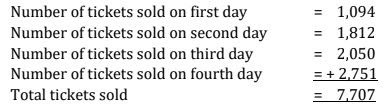Therefore, 7,707 tickets were sold on all the four days.

Question 2: Shekhar is a famous cricket player. He has so far scored 6980 runs in test matches. He wishes to complete 10,000 runs. How many more runs does he need?

Solution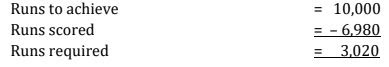Therefore, he needs 3,020 more runs.

Question 3: In an election, the successful candidate registered 5,77,500 votes and his nearest rival secured 3,48,700 votes. By what margin did the successful candidate win the election?

Solution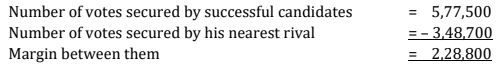Therefore, the successful candidate won by a margin of 2,28,800 votes.

Question 4: Kirti Bookstore sold books worth ₹2,85,891 in the first week of June and books worth ₹4,00,768 in the second week of the month. How much was the sale for the two weeks together? In which week was the sale greater and by how much?

Solution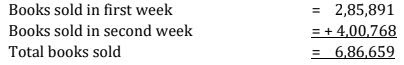Since, 4,00,768,> 2,85,891

Therefore sale of second week is greater than that of first week.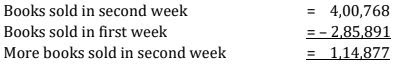Therefore, 1,14,877 more books were sold in second week.

Question 5: Find the difference between the greatest and the least number that can be written using the digits 6, 2, 7, 4, 3 each only once.

Solution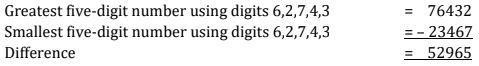Therefore the difference is 52965.

Question 6: A machine, on an average, manufactures 2,825 screws a day. How many screws did it produce in the month of January 2006?

Solution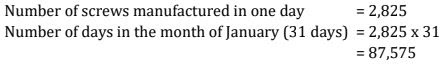Therefore, the machine produced 87,575 screws in the month of January.

Question 7: A merchant had ₹78,592 with her. She placed an order for purchasing 40 radio sets at ₹1,200 each. How much money will remain with her after the purchase?

Solution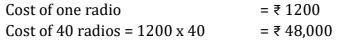Now,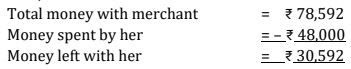Therefore, ₹ 30,592 will remain with her after the purchase.

Question 8: A student multiplied 7236 by 65 instead of multiplying by 56. By how much was his answer greater than the correct answer?

Solution

Wrong answer = 7236 × 65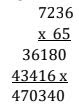Correct answer = 7236 × 56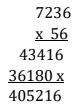Difference in answers = 470340 – 405216 = 65,124

Question 9: To stitch a shirt 2 m 15 cm cloth is needed. Out of 40 m cloth, how many shirts can be stitched and how much cloth will remain?

Solution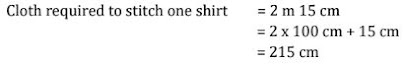Length of cloth = 40 m = 40 × 100 cm = 4000 cm

Number of shirts can be stitched = 4000/215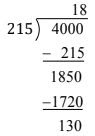Therefore, 18 shirts can be stitched and 130 cm (1 m 30 cm) cloth will remain.

Question 10: Medicine is packed in boxes, each weighing 4 kg 500 g. How many such boxes can be loaded in a can which cannot carry beyond 800 kg?

Solution

The weight of one box = 4 kg 500 g = 4 × 1000 g + 500 g = 4500 g

Maximum load can be loaded in van = 800 kg = 800 × 1000 g = 800000 g

Number of boxes = 800000/4500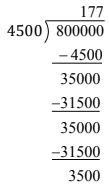Therefore, 177 boxes can be loaded.

Question 11: The distance between the school and the house of a student’s house is 1 km 875 m. Every day she walks both ways. Find the total distance covered by her in six days.

Solution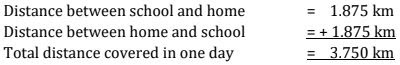Distance covered in six days = 3.750 × 6 = 22.500 km

Therefore, 22 km 500 m distance covered in six days.

Question 12: A vessel has 4 litres and 500 ml of curd. In how many glasses each of 25 ml capacity, can it be filled?

Solution

Capacity of curd in a vessel = 4 litres 500 ml = 4 × 1000 ml + 500 ml = 4500 ml

Capacity of one glass = 25 ml

Number of glasses can be filled = 4500/25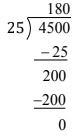Therefore, 180 glasses can be filled by curd.

Exercise 1.3

Question 1: Estimate each of the following using general rule:

(a) 730 + 998

(b) 796 – 314

(c) 12,904 + 2,888

(d) 28,292 – 21,496

Solution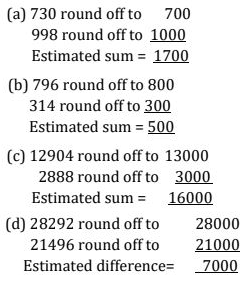Question 2: Give a rough estimate (by rounding off to nearest hundreds) and also a closer estimate (by rounding off to nearest tens):

(a) 439 + 334 + 4317

(b) 1,08,737 – 47,599

(c) 8325 – 491

(d) 4,89,348 – 48,365

Solution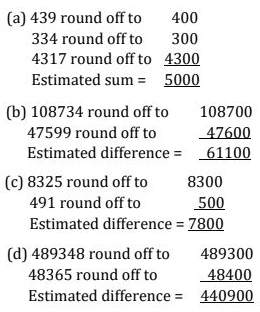Question 3: Estimate the following products using general rule:

(a) 578 × 161

(b) 5281 × 3491

(c) 1291 × 592

(d) 9250 × 29

Solution

(a) 578 × 161

578 round off to 600

161 round off to 200

The estimated product = 600 × 200 = 1,20,000

(b) 5281 × 3491

5281 round of to 5,000

3491 round off to 3,500

The estimated product = 5,000 × 3,500 = 1,75,00,000

(c) 1291 × 592

1291 round off to 1300

592 round off to 600

The estimated product = 1300 × 600 = 7,80,000

(d) 9250 × 29

9250 round off to 10,000

229 round off to 30

The estimated product = 10,000 × 30 = 3,00,000